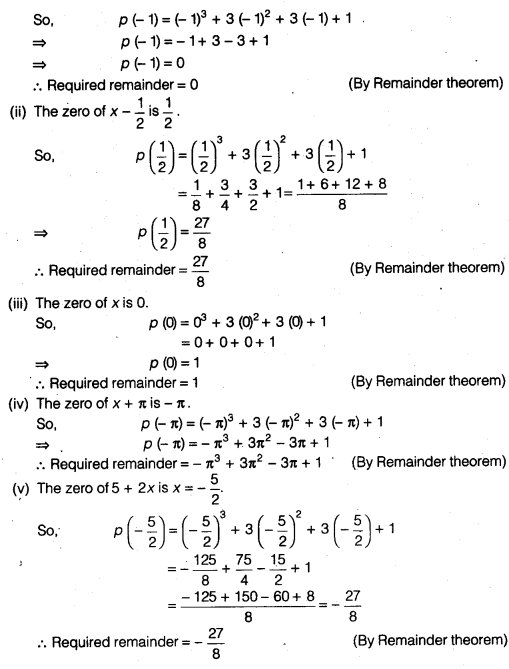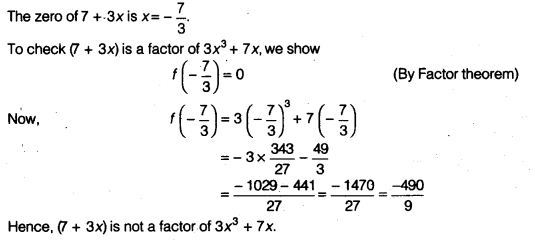NCERT Solutions for Class 9 Maths Chapter 2 Polynomials Ex 2.3 are part of NCERT Solutions for Class 9 Maths. Here we have given NCERT Solutions for Class 9 Maths Chapter 2 Polynomials Ex 2.3.

 Board CBSE Textbook NCERT Class Class 9 Subject Maths Chapter Chapter 2 Chapter Name Polynomials Exercise Ex 2.3 Number of Questions Solved 3 Category NCERT Solutions

## NCERT Solutions for Class 9 Maths Chapter 2 Polynomials Ex 2.3

Question 1.
Find the remainder when x3 + 3x2 + 3x + 1 is divided by
(i) x + 1
(ii) x – $$\frac { 1 }{ 2 }$$
(iii) x
(iv) x + π
(v) 5 + 2x
Solution:
Let p (x) = x3 + 3x2 + 3x + 1
(i) The zero of x+ 1 is – 1. (∵ x+ 1 = 0= x = -1)Question 2.
Find the remainder when x3-ax2 +6x-ais divided by x – a.
Solution:
The zero of x – a is a. (∵ x – a = 0 =» x= a)
Let p (x) = x3 – ax2 + 6x – a
So, p (a) = a3 – a (a)2 + 6a – a = a3 – a3 + 5a
⇒ p (a) = 5a
∴ Required remainder = 5a (By Remainder theorem)

Question 3.
Check whether 7 + 3x is a factor of 3x3+7x.
Solution:
Let f(x) = 3x3+7xWe hope the NCERT Solutions for Class 9 Maths Chapter 2 Polynomials Ex 2.3, help you. If you have any query regarding NCERT Solutions for Class 9 Maths Chapter 2 Polynomials Ex 2.3, drop a comment below and we will get back to you at the earliest.